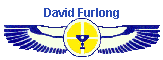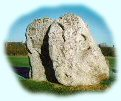Earth Mysteries Keys to Temple Introduction Keys to Temple Pt 1 - The British Pyramid Keys to Temple Pt 2 - The British Pyramid Sekeds and      Pyramid Geometry 666 - A magic number? Whatever happened in 3100 bc? Avebury's          Sacred Geometry Who were the Elohim? The Cotswold Circle Marlborough Downs Long Barrow Mystery   (coming soon) Main Selection Home
Sekeds and the Geometry of the
Egyptian Pyramids
article by David Furlong

A comparison between the angles generated by sekeds
and the angles of gradient of the pyramids

Part 5Pi and the cubit

The most simple ratio for the pi (ã) formula is 22/7, although today it is usually expressed decimally as 3.1416. Using the first of these two equations it becomes obvious that a circle with a radius of 7 units has a circumference of 44 units. In other words a circle with a radius of 1 cubit has a circumference of 44 palms. Using this method it is very easy to sub-divide the circumference into halves, quarters, eighths and sixteenths; there being 22 palms in a semi-circle, 11 palms in a quadrant and 5.5 palms in 1/8th of circle and 2.75 palms in 1/16th. In addition it is also very easy, through simple multiplication or division, to arrive at length of the circumference or a circle, or part thereof, once the radius is known. For example a radius of 2 cubits gives a circumference of 88 palms; 1.5 cubits has a circumference of 66 palms and so on.

There is no evidence in the mathematical texts that the Ancient Egyptians knew the 'pi' formula as such. In practice this would not be necessary for the formula was already contained within their measuring system through the simple adoption of the sevenfold division of the cubit.

Seen in this light it now becomes apparent why the Egyptians might have adopted a seked of 5.5 [5 palms and 2 digits] for the Great Pyramid and at least two other pyramids. As has often been stated the distance around the base of the Great Pyramid exactly equals the circumference of a circle whose radius is the height of the pyramid. Through the ratio of 7:11 there is a direct relationship between the three main elements in architecture, the square, the triangle and the circle. In the case of the Great Pyramid the circle is unseen, which equates with how it is often perceived in mystical tradition representing infinity, or the element of the divine.

In further support of these arguments the cubit measures of the Great Pyramid are generally given as base sides being 440 cubits and the height as 280 cubits, which are directly related to the numeric ratios stated above. The two numbers 7 and 11 can also be found woven into other elements of the pyramid's design. For example the height of the King's Chamber is 11 cubits and there are 7 corbels to each side of the Grand Gallery. These elements may have been coincidental being applied for practical or aesthetic reasons. It is also possible that they may have contained a symbolic function in relation to the numeric ratios contained within the pyramids design.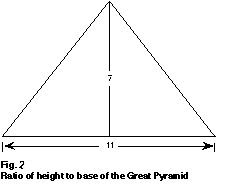.Seked problems

Having considered reasons why seked's of 5.25 and 5.5 might have been used, there is still the problem of those pyramids which do not easily fit with the known seked ratios. These are the pyramids of Menkaure (51.19°), the Northern Stone Pyramid (43.6°) and the upper part of the Bent Pyramid (43.35°).

In examining these anomalies I first wish to focus on the Northern Stone Pyramid of Sneferu. Slight variations in the angle of slope are given by different authorities. For example I. E. S. Edwards gives an angle of 43°-36', whilst Mark Lehner in his book The Complete Pyramids suggests 43°-22' and Grinsell in his book Egyptian Pyramids 8gives 43°-40'. It should be remembered that accurate determination of the angle is difficult, although in the case of relatively intact pyramids, of which this is one, the best assessment can be made from measuring the length of the base and the size and height above base level of the highest remaining course. This gives reasonably accurate figures from which to calculate the angle of slope.

In a recent article by George Johnson, in KMT magazine (Vol. 8, no. 3), the angle of slope of the Northern Pyramid is given as 43°-36'-11", with an original base of 722 feet and a height of 343 feet. If these measurements are correct then the angle of slope can be shown to be 43° - 32' - 7". However the angle given suggests that the height measurement may not be completely accurate. To precisely fit an angle of 43° -36' -11" the height would need to be 343.812 feet.

This distances can be compared with the standard Egyptian measure of a Royal cubit. The base sides can be shown to be 420 cubits derived from a cubit length of 1.719 feet. Dividing this ratio into the height gives a figure of exactly 200 cubits (343.8 / 1.1719 = 200). The height to base ratio can therefore be shown to be 20:21 which conforms to the ratio figure given by Petrie. However this ratio does not fit a standard seked giving a measure of 7.35 sekeds. As already stated it, would not be practical, in constructional terms, to work with a seked based on a fraction of a digit.

All authorities suggest angles that fall around forty-three and a half degrees. This needs to be compared with sekeds of 7.25 which gives an angle of 43.99° and 7.5 which produces 43.02°. By way of comparison a pyramid with a base of 722 feet and a seked of 7.25 would have a height of 348.55 feet, whilst one with a seked of 7.5 would be 336.93 feet. Of these two measures 348.55 lies closest to the presumed height of 343.81 feet. However the discrepancy of 4.75 feet is greater than one would expect from an accurate survey. All of this evidence suggests that, on occasions, the Ancient Egyptians used different ratios for calculating their seked.

This situation is further highlighted in the Bent Pyramid which also has an upper angle of around 43.5°. The angle of the lower half of this pyramid (54.52°) falls within 3.6' of a seked of 5.00 (54.46°), whilst the upper portion is nearly one third of a degree adrift from a seked of 7.5. If we assume that the measurements of this pyramid are accurate, which the seked for the lower portion suggests, then we are forced to the conclusion that variations for seked calculations must have occurred, despite the lack of textual evidence in RMP.

The ratio of 20:21 can be derived if the one cubit height is reduced by 5/7ths, which can be restated as working to 20 palms height rather than 28 for a full cubit.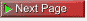or full article download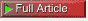or text only articlePage 1, 2, 3, 4, 5, 6

Home | Keys | Profile | Tours | Courses | Books | Articles | Calendar | Contact | Links